## Ellipse EnvelopeConsider the family of Ellipses(1)

for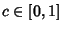. The Partial Derivative with respect to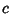is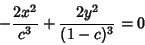(2)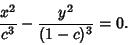(3)

Combining (1) and (3) gives the set of equations(4)(5)

where the Discriminant is(6)

so (5) becomes(7)

Eliminatingthen gives(8)

which is the equation of the Astroid. If the curve is instead represented parametrically, then(9)(10)

Solving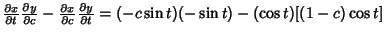(11)
forgives(12)

so substituting this back into (9) and (10) gives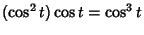(13)(14)

the parametric equations of the Astroid.